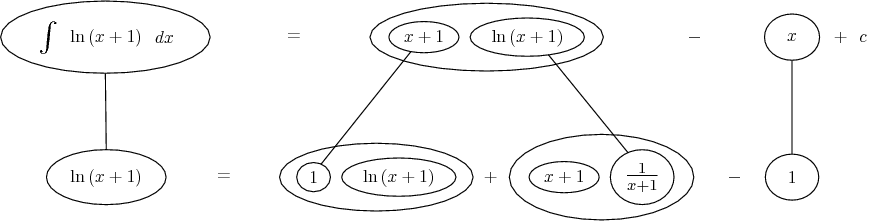# Thread: integration by parts

1. ## integration by parts

use integration by parts to find the integral of ln(x+1) dx
any help would be appreciated

2. $\displaystyle \int ln(1+x)dx=\int1.ln(1+x)dx$

Let $\displaystyle u=ln(1+x)$ and $\displaystyle dv=1$

Then $\displaystyle du=\frac{1}{1+x}$ and $\displaystyle v=x$

$\displaystyle xln(1+x)- \int x \frac{1}{1+x}dx=xln(1+x)- \int 1dx=xln(1+x)-x+C$

3. Don't forget the absolute value.4. how does the integral of x/(x+1) become the integral of 1??
thanks

5. because when you let $\displaystyle u=x$ and $\displaystyle dv=\frac{1}{1+x}=(1+x)^{-1}$, $\displaystyle du=1$ and $\displaystyle v=(1+x)^0=1$

6. Whoah! Careful now.

You could split

$\displaystyle \frac{x}{x+1}$

into

$\displaystyle 1 - \frac{1}{x+1}$

and have

$\displaystyle I = x\ \ln(x+1) - \int (\ 1 - \frac{1}{1+x}\ )\ dx$

$\displaystyle \ \ =x\ \ln(1+x) - x + \ln(x+1)+C$

which is fine.

But here's a short cut - notice that one integral of 1 is x + 1, and choose u = x+1 for du = 1.

I.e.

$\displaystyle I = (x+1)\ \ln(x+1) - \int (x+1)\ \frac{1}{x+1}\ dx$

$\displaystyle = (x+1)\ \ln(x+1) - \int (1)\ dx$

$\displaystyle \ \ = (x+1)\ \ln(x+1) - x + C$

Picture shortly...

7.Don't integrate - balloontegrate!

Balloon Calculus: worked examples from past papers

#### Search Tags

integration, parts